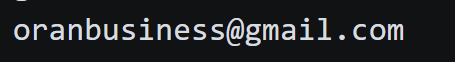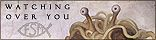# Data visualization: How math terms relate to each other

I made a graph of a big list of math terms and used Wikipedia's links to relate them dynamically itperfects not perfict but it sure is beautiful!

I made a graph of a big list of math terms and used Wikipedia's links to relate them dynamically itperfects not perfict but it sure is beautiful!

Note: the app is made by this person Xefer link found here

# Lets Look a bit closer on some things:

💡
Take a look at `convex set`

#### what this graph reveals is `informational grouping` about data.

for example:

I don't know what convex set is but I do know what geometry is and what Arithmetic means so I can guess by the structure its something to do with geometric math stuff

## Ambiguity

### Where it breaks down

💡
heres a good example of where the word ex: Identity is very ambigus, and could be interated to mean alot of things

## I think that this model of mapping human understanding to a graph tree structure is a great way for computers to get a good understanding of human language

💡
IDEA: have the algorithm that does create the graph structure automatically when receiving data, alot like what humans do when were learning something new

## Play with the graph for yourself

### 2) paste this big string into the search box and click `submit`

``abstract algebra, algebra, algebraic expression, algebraic equation, algorithm, amicable numbers, analytic (Cartesian) geometry, analysis (mathematical analysis), arithmetic, associative property, asymptote, axiom, base n, Bayesian probability, bell curve, bijection, binomial, binomial coefficients, Boolean algebra or logic, calculus (infinitesimal calculus), calculus of variations, cardinal numbers, Cartesian coordinates, coefficients, combinatorics, complex dynamics, complex number, composite number, congruence, conic section, continued fraction, coordinate, coordinate plane, correlation, cubic equation, decimal number, deductive reasoning or logic, derivative, descriptive geometry, differential equation, differential geometry, differentiation, Diophantine equation, distributive property, element, ellipse, elliptic geometry, empty (null) set, Euclidean geometry, expected value, exponentiation, factor, factorial, Fermat primes, Fibonacci numbers (series), finite differences, formula, Fourier series, fraction, fractal, function, game theory, Gaussian curvature, geometry, golden ratio (golden mean, divine proportion), graph theory, group, group theory, Hilbert problems, hyperbola, hyperbolic geometry, identity, imaginary numbers, inductive reasoning or logic, infinite series, infinitesimal, infinity, integers, integral, integration, irrational numbers, Julia set, knot theory, least squares method, limit, line, linear equation, linear regression, logarithm, logic, logicism, magic square, Mandelbrot set, manifold, matrix, Mersenne number, Mersenne primes, method of exhaustion, modular arithmetic, modulus, monomial, natural numbers, negative numbers, non-commutative algebra, non-Euclidean geometry, normal (Gaussian) distribution, number line, number theory, ordinal numbers, parabola, paradox, partial differential equation, Pascal’s Triangle, perfect number, periodic function, permutation, pi (π), place value, Platonic solids, polar coordinates, polynomial, prime numbers, projective geometry, plane, probability theory, Pythagoras’ (Pythagorean) theorem, Pythagorean triples, quadratic equation, quadrature, quartic equation, quaternions, quintic equation, rational numbers, real numbers, reciprocal, Riemannian geometry, right triangle, self-similarity, sequence, set, significant digits, simultaneous equations, slope, spherical geometry, spherical trigonometry, subset, surd, symmetry, tensor, term, theorem, topology, transcendental number, transfinite numbers, triangular number, trigonometry, trinomial, type theory, vector, vector space, Venn diagram, Zermelo-Fraenkel set theory, Zeta function``

source: https://www.storyofmathematics.com/glossary.html but stripped and cleaned up a bit with one of my projects Text Processor

### and....Boom done!# Author

by oran collins
github.com/wisehackermonkeyIf you want to help me out and give some donations here's my monero address: 432ZNGoNLjTXZHz7UCJ8HLQQsRGDHXRRVLJi5yoqu719Mp31x4EQWKaQ9DCQ5p2FvjQ8mJSQHbD9WVmFNhctJsjkLVHpDEZ I use a tracker that is pravicy focused so if you block its cool, im big on blocking stuff on my own machine. im doing it to see if anyone is actualy reading my blog posts...:)